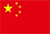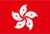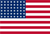Scanning QR Code• Online Inquiry

+
Congratulations
You've successfully got advanced quotes worth 5000 HKD
• Home
• Quotes
• News
• Learn
• Help Center
• Language
•••EN

# Risk indicator description

### 1. α (Alpha)

The Alpha coefficient is the difference between the actual income of the fund and the expected return calculated on the basis of the β coefficient. The calculation method is as follows: excess return is the income of the fund minus the return of venture capital (in China, it is the income of time deposit of one-year bank); the expected income is the product of beta coefficient β and market income, which reflects the income obtained by the fund as a result of the overall change in the market; and the difference between excess return and expected return is the α coefficient.

### 2. β (Beta)

Beta coefficient is a relative index to measure the overall volatility of fund return relative to the benchmark return of performance evaluation. The higher the β, the greater the volatility of the fund relative to the performance evaluation benchmark. If β is greater than 1, the volatility of the fund is greater than that of the performance evaluation benchmark. And vice versa. If β is 1, the market is up 10% and the fund is up 10%. The market is down 10%, and the fund is down 10%.

### 3. R Square (R-squared)

R Square (R-squared) is the impact of changes in performance benchmark on the performance of the Fund, with an impact of 0 to 100. If the R square value is equal to 100, the change in the return of the fund is entirely due to a change in the performance benchmark; if the R square value is equal to 35, 35 per cent of the return of the fund can be attributed to a change in the performance benchmark. In short, the lower the R square value, the less changes in the performance of the Fund as a result of changes in the performance benchmark.

### 4. Sharp (Sharpe Ratio)

Sharp (Sharpe Ratio) is one of the indexes to measure the adjusted return of fund risk, which reflects that the excess rate of return ((Excess Returns),) obtained by the unit risk of the fund is higher than that of the risk-free rate of return of the fund in the same period. In general, the higher the ratio, the higher the excess rate of return of the unit risk of the fund.

### 5. Standard deviation (Standard Deviation)

The standard deviation (Standard Deviation) reflects the fluctuation range of the total rate of return during the calculation period, that is, the deviation degree of the total rate of return of the fund compared with the average monthly rate of return, the greater the fluctuation, the greater the standard deviation.

### 6. Tracking error (Tracking Error)

Tracking error (Tracking Error) refers to the standard deviation of return between the combination rate of return and the benchmark rate of return (market index rate of return), which reflects the risk of fund management. The larger the tracking error, the greater the difference between the net value ratio of the fund and the return rate of the benchmark portfolio, and the greater the risk of the active investment of the fund manager.

### 7. Information ratio (Information Ratio)

Information Ratio is a risk-adjusted excess return that measures a portfolio better than a particular index. The larger the information ratio, the higher the excess return obtained by the tracking error of the fund manager unit. Therefore, the performance of the fund with large information ratio is better than that of the fund with lower information ratio.

### 8. Traineau ratio (Treynor Ratio)

Treynor Ratio is the risk premium per unit risk, and it is the index for investors to judge whether the risk taken by a fund manager in the process of managing the fund is beneficial to the investor. The larger the Traineau index, the higher the unit risk premium, the better the performance of open-end funds, and the risk taken by fund managers in the process of management is conducive to the profits of investors. On the contrary, the smaller the Traineau index, the lower the unit risk premium, the worse the performance of open-end funds, and the risks taken by fund managers in the process of management are not conducive to the profits of investors.

### 9. Up / down catch rate (Capture Ratio Upside / Downside)

Capture Ratio refers to the performance of fund managers in the market, which is generally used to evaluate the performance of fund managers. The up / down capture ratio can show whether a given fund outperformed the market benchmark during a period of market strength and, if so, the increase was smaller than the market benchmark. The uplink capture rate of the fund is obtained by dividing the monthly fund return in the month in which the benchmark month is positive by the benchmark rate of return for the month. The downlink capture ratio of the Fund is calculated by dividing the monthly return for the period when the benchmark performance is negative by the benchmark return. The formula is: Up/Down-Market Capture Ratio= (Manager's Returns/Index Returns) * 100An upward capture rate of more than 100 suggests that a fund usually outperforms the benchmark during periods when benchmark earnings are positive. At the same time, a downward capture rate of less than 100 indicates that the Fund's losses are lower than its benchmark at a benchmark loss. However, if a fund produces positive returns and the benchmark index falls, the fund's downlink capture ratio will be negative (which means it has moved in the opposite direction of the benchmark). The ratio of rise and fall of all equity funds to the S & P 500 index is calculated, while the ratios of bonds and international funds are calculated relative to the Barclays Capital US Total Bond Index and the MSCI EAFE Index, respectively.

### 10. Average hit rate (Batting Average)

Batting Average is used to measure the ability of fund managers to reach or exceed the index. The highest average may be 100 per cent, indicating that fund managers outperform the benchmark at each period.

### 11. Sotino ratio (Sortino Ratio)

Sortino Ratio is similar to Sharp ratio in that it distinguishes between good or bad fluctuations, so it uses not standard deviation, but downlink standard deviation, in calculating volatility. The implicit condition is that the rise in the portfolio (positive rate of return) is in line with the needs of investors and should not be included in the risk adjustment.The higher the ratio, the higher the excess rate of return for the fund to take on the same downside risks per unit. The Sotino ratio can be seen as a correction of the Sharp ratio when measuring hedge funds / private equity funds.

### 12. Kurtosis (Kurtosis)

Kurtosis (peakedness;kurtosis) is also known as peak state coefficient. The characteristic number of the peak value of the probability density distribution curve at the average value. Intuitively, the kurtosis reflects the cusp of the peak. The kurtosis of the sample is compared with the normal distribution and measured by statistics. If the kurtosis is more than three, the shape of the peak is sharper and steeper than the normal distribution peak. And vice versa. In statistics, kurtosis (Kurtosis) measures the peak state of probability distribution of real random variables. High kurtosis means that the increase of variance is caused by the extreme difference of low frequency greater than or less than the average.

### 13. Skewness (Skewness)

Skewness (skewness), is a measure of the skew direction and degree of statistical data distribution, and it is the digital feature of the asymmetrical degree of statistical data distribution. Skewness (Skewness) is also called skewness and skewness coefficient. The characteristic number that represents the asymmetry degree of the probability distribution density curve relative to the average value. Intuitively, it is the relative length of the end of the density function curve.

Back to the Top